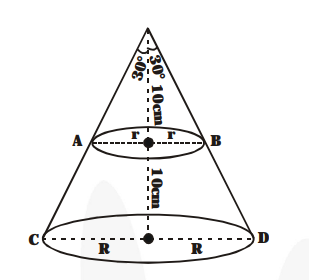# A metallic right circular cone 20 cm high and whose vertical angle is 60° is cut into two parts at the middle of its height by a plane parallel to its base.

Question:

A metallic right circular cone 20 cm high and whose vertical angle is 60° is cut into two parts at the middle of its height by a plane parallel to its base. If the frustum so obtained be drawn into a wire of diameter 1/16 cm, find the length of the wire.

Solution:

From the figure$\frac{\mathbf{R}}{\mathbf{2 0}}=\tan 30^{\circ}=\frac{\mathbf{1}}{\sqrt{3}}$, i.e., $\mathrm{R}=\frac{\mathbf{2 0}}{\sqrt{\mathbf{3}}} \mathrm{cm}$

$\frac{\mathbf{r}}{\mathbf{1 0}}=\tan 30^{\circ}=\frac{\mathbf{1}}{\sqrt{\mathbf{3}}}$, i.e., $\mathrm{r}=\frac{\mathbf{1 0}}{\sqrt{\mathbf{3}}} \mathrm{cm}$

h = 10 cm is the height of the frust.

Volume of the material in the frustum ACDB

$=\frac{\mathbf{1}}{\mathbf{3}} \pi \times \mathrm{h} \times\left\{\mathrm{R}^{2}+\mathrm{r}^{2}+\mathrm{Rr}\right\}$

$=\frac{1}{3} \pi \times 10 \times\left\{\frac{400}{3}+\frac{100}{3}+\frac{200}{3}\right\} \mathrm{cm}^{3}$

$=\frac{\mathbf{7 0 0 0}}{\mathbf{9}} \pi \mathbf{c m}^{3}$

Now let us suppose wire of diameter $\frac{\mathbf{1}}{\mathbf{1 6}} \mathrm{cm}$ is made of length $x \mathrm{~cm}$.

Then, $\pi \times\left(\frac{1}{32}\right)^{2} \times x=\frac{7000}{9} \pi$

$x=\frac{7000 \times 32 \times 32}{9} \mathrm{~cm}$

$x=\frac{\mathbf{7 1 6 8 0}}{\mathbf{9}} \mathrm{m}=7964.4 \mathrm{~m}$